# Non Verbal Reasoning - Analytical Reasoning

Exercise : Analytical Reasoning - Section 1
6.

Find the minimum number of straight lines required to make the given figure.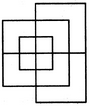13
15
17
19
Explanation:

The figure may be labelled as shown.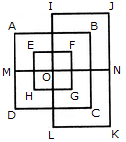The horizontal lines are IJ, AB, EF, MN, HG, DC and LK i.e. 7 in number.

The vertical lines are AD, EH, IL, FG, BC and JK i.e. 6 in number.

Thus, there are 7 + 6 = 13 straight lines in the figure.

7.

Find the number of triangles in the given figure.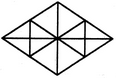16
22
28
32
Explanation:

The figure may be labelled as shown.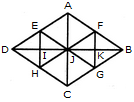The simplest triangles are AFJ, FJK, FKB, BKG, JKG, JGC, HJC, HIJ, DIH, DEI, EIJ and AEJ i.e. 12 in number.

The triangles composed of two components each are JFB, FBG, BJG, JFG, DEJ, EJH, DJH and DEH i.e. 8 in number.

The triangles composed of three components each are AJB, JBC, DJC and ADJ i.e. 4 in number.

The triangles composed of six components each are DAB, ABC, BCD and ADC i.e. 4 in number.

Thus, there are 12 + 8 + 4 + 4 = 28 triangles in the figure.

8.

Find the number of triangles in the given figure.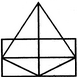11
13
15
17
Explanation:

The figure may be labelled as shown.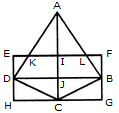The simplest triangles are AKI, AIL, EKD, LFB, DJC, BJC, DHC and BCG i.e. 8 in number.

The triangles composed of two components each are AKL, ADJ, AJB and DBC i.e. 4 in number.

The triangles composed of the three components each are ADC and ABC i.e. 2 in number.

There is only one triangle i.e. ADB composed of four components.

Thus, there are 8+ 4 + 2 + 1= 15 triangles in the figure.

9.

Find the number of triangles in the given figure.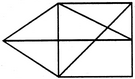12
13
14
15
Explanation:

The figure may be labelled as shown.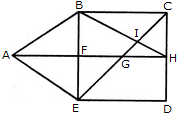The simplest triangles are ABF, BIC, CIH, GIH, FGE and AFE i.e. 6 in number.

The triangles composed of two components each are ABE, AGE, BHF, BCH, CGH and BIE i.e. 6 in number.

The triangles composed of three components each are ABH, BCE and CDE i.e. 3 in number.

Hence, the total number of triangles in the figure = 6 + 6 + 3 = 15.

10.

Find the number of triangles in the given figure.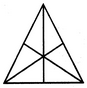16
13
9
7
Explanation:

The figure may be labelled as shown.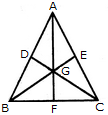The simplest triangles are AGE, EGC, GFC, BGF, DGB and ADG i.e. 6 in number.

The triangles composed of two components each are AGC, BGC and ABG i.e. 3 in number.

The triangles composed of three components each are AFC, BEC, BDC, ABF, ABE and DAC i.e. 6 in number.

There is only one triangle i.e. ABC composed of six components.

Thus, there are 6 + 3 + 6 + 1 = 16 triangles in the given figure.